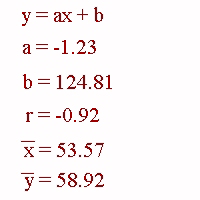### CFA Practice Question

There are 985 practice questions for this topic.

### CFA Practice Question

If you run linear regression on a set x-y data and obtain the results shown at the right then the linear regression line must pass through the point (______, ______).A. (124.81, -1.23)
B. (53.57, 58.92)
C. (-1.23, -0.92)